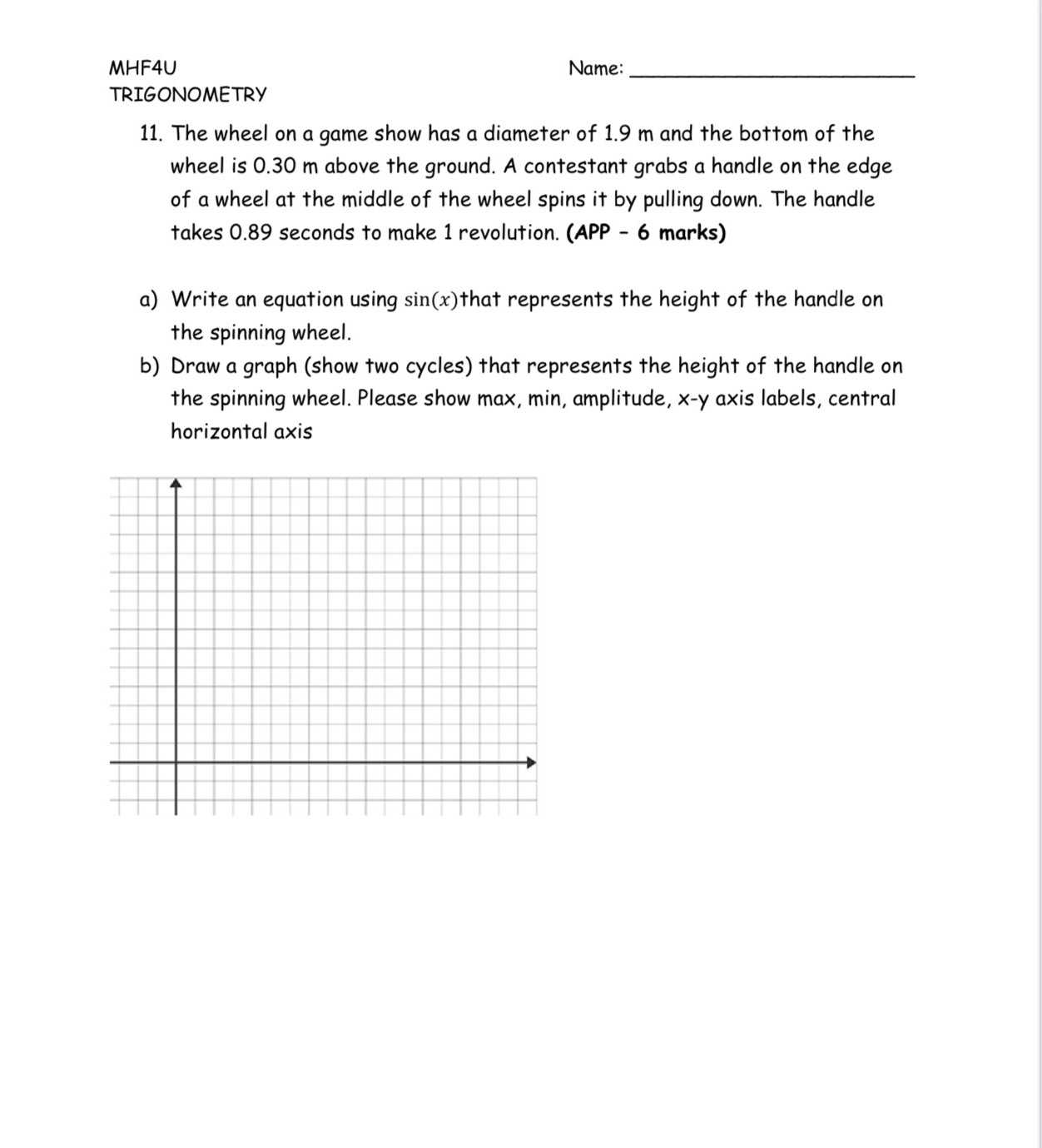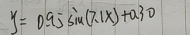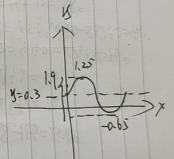### ¿Todavía tienes preguntas de matemáticas?

Pregunte a nuestros tutores expertos
Algebra
Pregunta11. The wheel on a game show has a diameter of $$1.9$$ m and the bottom of the wheel is $$0.30$$ m above the ground. A contestant grabs a handle on the edge of a wheel at the middle of the wheel spins it by pulling down. The handle takes $$0.89$$ seconds to make $$1$$ revolution. (APP - $$6$$ marks) a) Write an equation using $$\sin ( x )$$ that represents the height of the handle on the spinning wheel. b) Draw a graph (show two cycles) that represents the height of the handle on the spinning wheel. Please show max, min, amplitude, $$x$$ -y axis labels, central horizontal axis